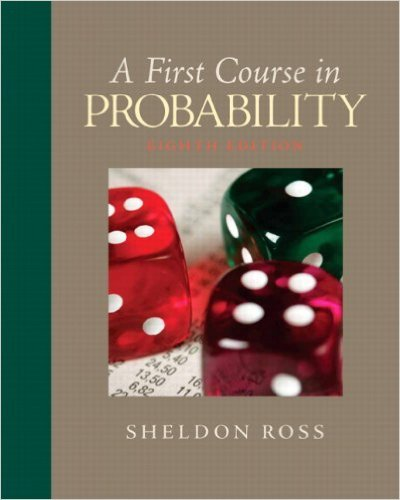×
Get Full Access to First Course In Probability - 8 Edition - Chapter 5 - Problem 5.10
Get Full Access to First Course In Probability - 8 Edition - Chapter 5 - Problem 5.10

×

# Trains headed for destination A arrive at the trainstationISBN: 9780136033134 163

## Solution for problem 5.10 Chapter 5

First Course in Probability | 8th Edition

• Textbook Solutions
• 2901 Step-by-step solutions solved by professors and subject experts
• Get 24/7 help from StudySoup virtual teaching assistantsFirst Course in Probability | 8th Edition

4 5 1 241 Reviews
18
2
Problem 5.10

Trains headed for destination A arrive at the trainstation at 15-minute intervals starting at 7 A.M.,whereas trains headed for destination B arrive at15-minute intervals starting at 7:05 A.M.(a) If a certain passenger arrives at the station ata time uniformly distributed between 7 and8 A.M. and then gets on the first train thatarrives, what proportion of time does he orshe go to destination A?(b) What if the passenger arrives at a time uniformlydistributed between 7:10 and 8:10A.M.?

Step-by-Step Solution:
Step 1 of 3

9/29/2017 STT 351 STT 351 Notes 2 Given a random variable, we can associate probability to subsets of real numbers Probability Model Tossing a coin, Rolling a die, Roulette wheel The experiment has random outcomes. We want to assign chance (probability) to subsets outcomes. S = totality of outcomes A = a subcollection of outcomes Associate P(A) probability for A Example: Toss a fair coin S = {H,T} A = {H} P({H}) = ½ Two assumptions: 1. Number of outcomes is finite 2. Each outcome has equal chance P({H}) = ½ Example: Rolling a die S = {1,2,3,4,5,6} A = even number of outcomes = {2,4,6}

Step 2 of 3

Step 3 of 3

##### ISBN: 9780136033134

Unlock Textbook Solution